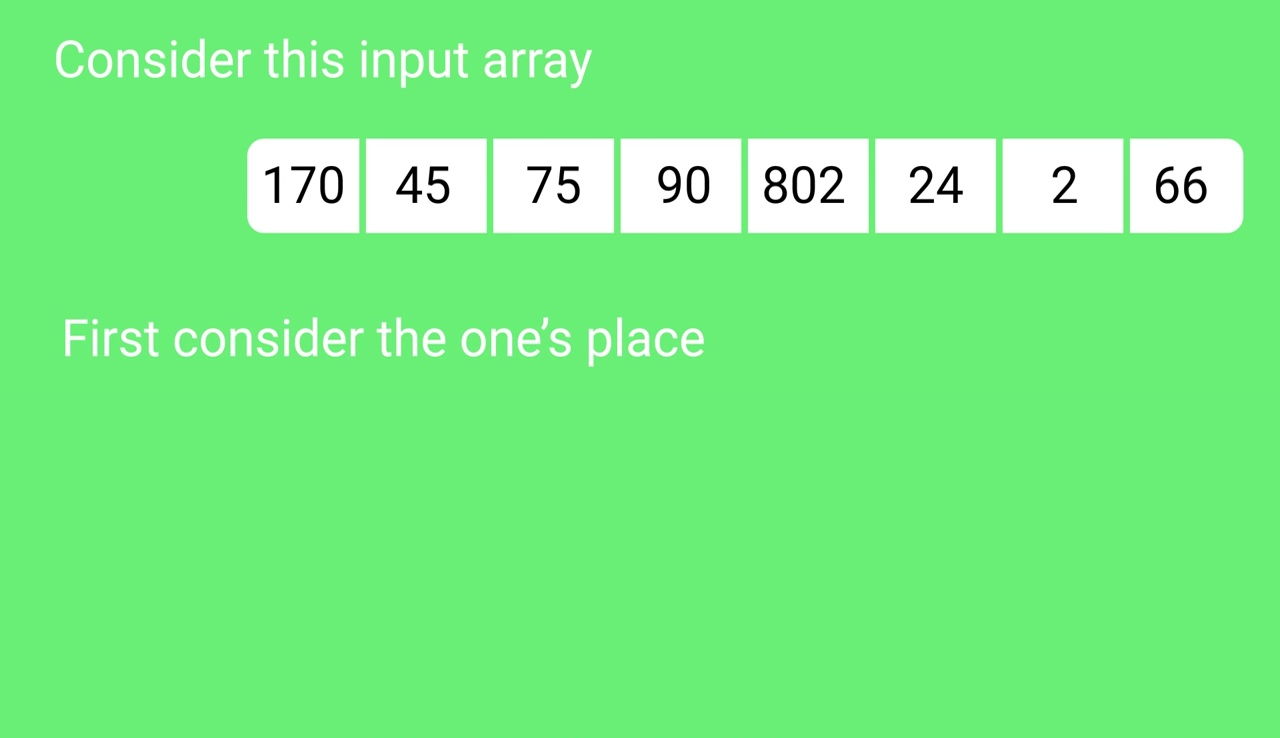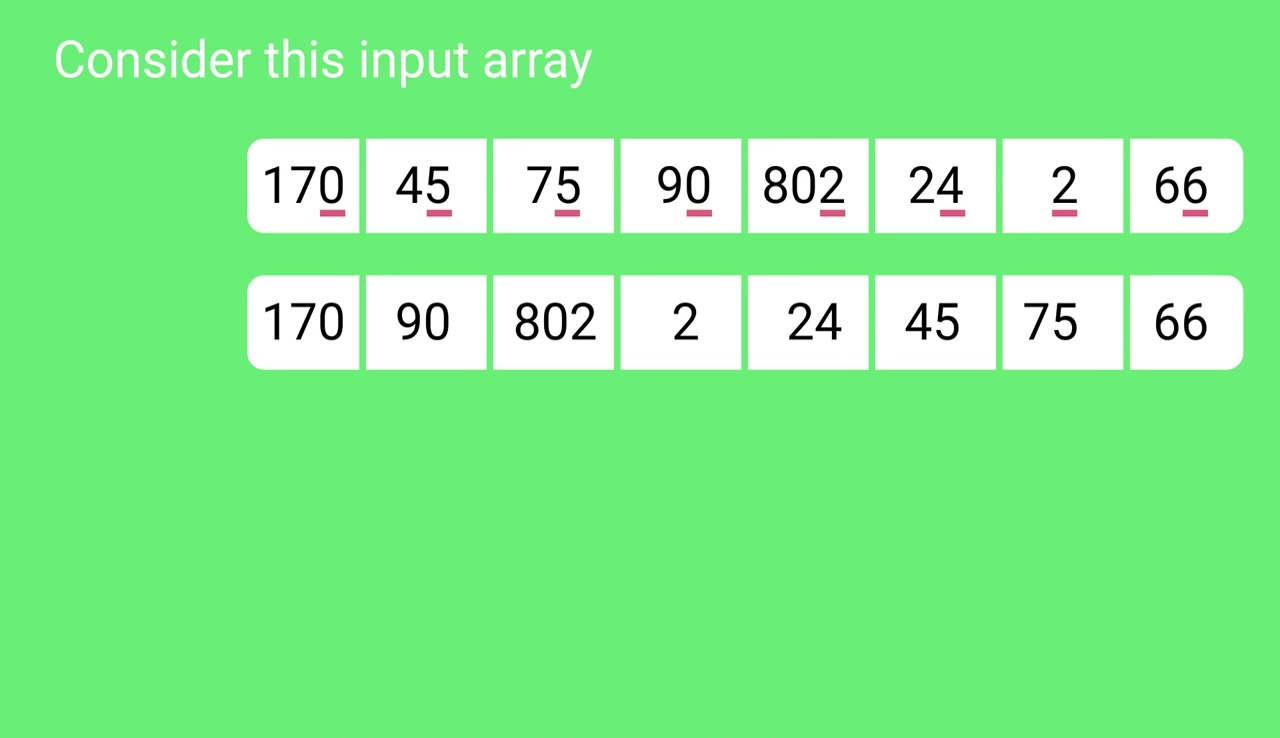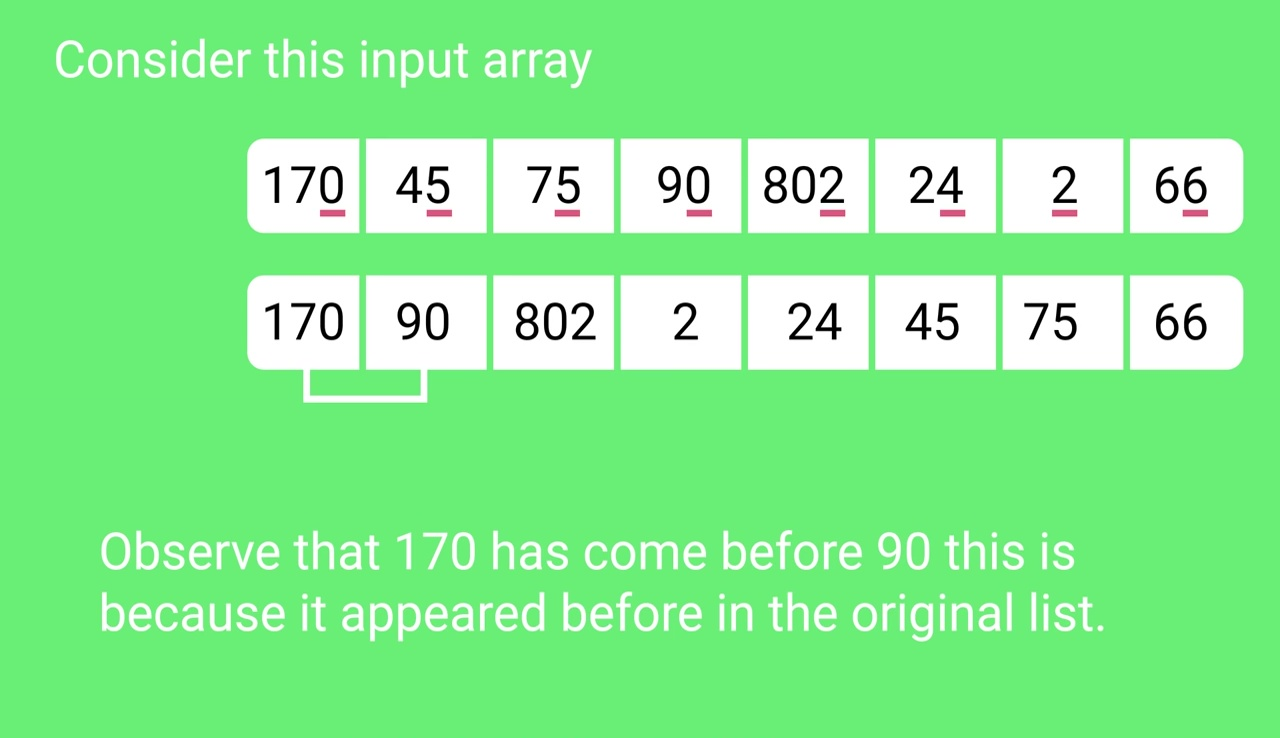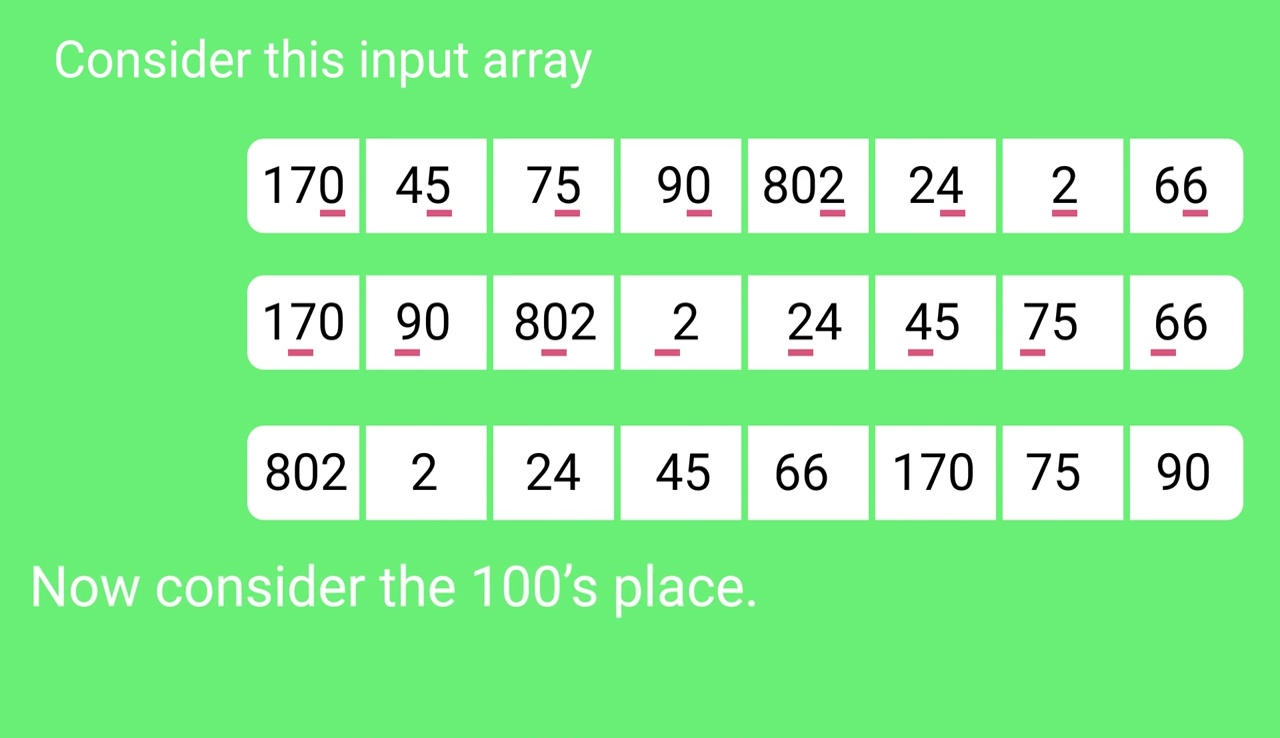• Difficulty Level : Medium
• Last Updated : 09 Aug, 2022

The lower bound for the Comparison based sorting algorithm (Merge Sort, Heap Sort, Quick-Sort .. etc) is Ω(nLogn), i.e., they cannot do better than nLogn. Counting sort is a linear time sorting algorithm that sort in O(n+k) time when elements are in the range from 1 to k.

What if the elements are in the range from 1 to n2

We can’t use counting sort because counting sort will take O(n2) which is worse than comparison-based sorting algorithms. Can we sort such an array in linear time?

Radix Sort is the answer. The idea of Radix Sort is to do digit by digit sort starting from least significant digit to most significant digit. Radix sort uses counting sort as a subroutine to sort.

Do the following for each digit I where I varies from the least significant digit to the most significant digit. Here we will be sorting the input array using counting sort (or any stable sort) according to the i’th digit.

Example:

Original, unsorted list: 170, 45, 75, 90, 802, 24, 2, 66 Sorting by least significant digit (1s place) gives: [*Notice that we keep 802 before 2, because 802 occurred before 2 in the original list, and similarly for pairs 170 & 90 and 45 & 75.] 170, 90, 802, 2, 24, 45, 75, 66 Sorting by next digit (10s place) gives: [*Notice that 802 again comes before 2 as 802 comes before 2 in the previous list.] 802, 2, 24, 45, 66, 170, 75, 90 Sorting by the most significant digit (100s place) gives: 2, 24, 45, 66, 75, 90, 170, 802

What is the running time of Radix Sort?

Let there be d digits in input integers. Radix Sort takes O(d*(n+b)) time where b is the base for representing numbers, for example, for the decimal system, b is 10. What is the value of d? If k is the maximum possible value, then d would be O(logb(k)). So overall time complexity is O((n+b) * logb(k)). Which looks more than the time complexity of comparison-based sorting algorithms for a large k. Let us first limit k. Let k <= nc where c is a constant. In that case, the complexity becomes O(nLogb(n)). But it still doesn’t beat comparison-based sorting algorithms.
What if we make the value of b larger? What should be the value of b to make the time complexity linear? If we set b as n, we get the time complexity as O(n). In other words, we can sort an array of integers with a range from 1 to nc if the numbers are represented in base n (or every digit takes log2(n) bits).

• In a typical computer, which is a sequential random-access machine, where the records are keyed by multiple fields radix sort is used. For eg., you want to sort on three keys month, day and year. You could compare two records on year, then on a tie on month and finally on the date. Alternatively, sorting the data three times using Radix sort first on the date, then on month, and finally on year could be used.
• It was used in card sorting machines with 80 columns, and in each column, the machine could punch a hole only in 12 places. The sorter was then programmed to sort the cards, depending upon which place the card had been punched. This was then used by the operator to collect the cards which had the 1st row punched, followed by the 2nd row, and so on.

Is Radix Sort preferable to Comparison based sorting algorithms like Quick-Sort?

If we have log2n bits for every digit, the running time of Radix appears to be better than Quick Sort for a wide range of input numbers. The constant factors hidden in asymptotic notation are higher for Radix Sort and Quick-Sort uses hardware caches more effectively. Also, Radix sort uses counting sort as a subroutine and counting sort takes extra space to sort numbers.

1. It makes assumptions about the data like the data must be between a range of elements.
2. Input array must have the elements with the same radix and width.
3. Radix sort works on sorting based on an individual digit or letter position.
4. We must start sorting from the rightmost position and use a stable algorithm at each position.
5. Radix sort is not an in-place algorithm as it uses a temporary count array.

FLowcharts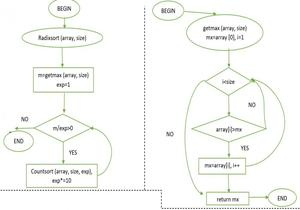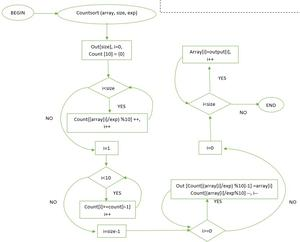Flowchart countsort

Following is a simple implementation of Radix Sort. For simplicity, the value of d is assumed to be 10. We recommend you to see Counting Sort for details of countSort() function in the below code.

## C++

 `// C++ implementation of Radix Sort` `#include ``using` `namespace` `std;` `// A utility function to get maximum value in arr[]``int` `getMax(``int` `arr[], ``int` `n)``{``    ``int` `mx = arr;``    ``for` `(``int` `i = 1; i < n; i++)``        ``if` `(arr[i] > mx)``            ``mx = arr[i];``    ``return` `mx;``}` `// A function to do counting sort of arr[] according to``// the digit represented by exp.``void` `countSort(``int` `arr[], ``int` `n, ``int` `exp``)``{``    ``int` `output[n]; ``// output array``    ``int` `i, count = { 0 };` `    ``// Store count of occurrences in count[]``    ``for` `(i = 0; i < n; i++)``        ``count[(arr[i] / ``exp``) % 10]++;` `    ``// Change count[i] so that count[i] now contains actual``    ``//  position of this digit in output[]``    ``for` `(i = 1; i < 10; i++)``        ``count[i] += count[i - 1];` `    ``// Build the output array``    ``for` `(i = n - 1; i >= 0; i--) {``        ``output[count[(arr[i] / ``exp``) % 10] - 1] = arr[i];``        ``count[(arr[i] / ``exp``) % 10]--;``    ``}` `    ``// Copy the output array to arr[], so that arr[] now``    ``// contains sorted numbers according to current digit``    ``for` `(i = 0; i < n; i++)``        ``arr[i] = output[i];``}` `// The main function to that sorts arr[] of size n using``// Radix Sort``void` `radixsort(``int` `arr[], ``int` `n)``{``    ``// Find the maximum number to know number of digits``    ``int` `m = getMax(arr, n);` `    ``// Do counting sort for every digit. Note that instead``    ``// of passing digit number, exp is passed. exp is 10^i``    ``// where i is current digit number``    ``for` `(``int` `exp` `= 1; m / ``exp` `> 0; ``exp` `*= 10)``        ``countSort(arr, n, ``exp``);``}` `// A utility function to print an array``void` `print(``int` `arr[], ``int` `n)``{``    ``for` `(``int` `i = 0; i < n; i++)``        ``cout << arr[i] << ``" "``;``}` `// Driver Code``int` `main()``{``    ``int` `arr[] = { 170, 45, 75, 90, 802, 24, 2, 66 };``    ``int` `n = ``sizeof``(arr) / ``sizeof``(arr);``    ` `      ``// Function Call``      ``radixsort(arr, n);``    ``print(arr, n);``    ``return` `0;``}`

## Java

 `// Radix sort Java implementation` `import` `java.io.*;``import` `java.util.*;` `class` `Radix {` `    ``// A utility function to get maximum value in arr[]``    ``static` `int` `getMax(``int` `arr[], ``int` `n)``    ``{``        ``int` `mx = arr[``0``];``        ``for` `(``int` `i = ``1``; i < n; i++)``            ``if` `(arr[i] > mx)``                ``mx = arr[i];``        ``return` `mx;``    ``}` `    ``// A function to do counting sort of arr[] according to``    ``// the digit represented by exp.``    ``static` `void` `countSort(``int` `arr[], ``int` `n, ``int` `exp)``    ``{``        ``int` `output[] = ``new` `int``[n]; ``// output array``        ``int` `i;``        ``int` `count[] = ``new` `int``[``10``];``        ``Arrays.fill(count, ``0``);` `        ``// Store count of occurrences in count[]``        ``for` `(i = ``0``; i < n; i++)``            ``count[(arr[i] / exp) % ``10``]++;` `        ``// Change count[i] so that count[i] now contains``        ``// actual position of this digit in output[]``        ``for` `(i = ``1``; i < ``10``; i++)``            ``count[i] += count[i - ``1``];` `        ``// Build the output array``        ``for` `(i = n - ``1``; i >= ``0``; i--) {``            ``output[count[(arr[i] / exp) % ``10``] - ``1``] = arr[i];``            ``count[(arr[i] / exp) % ``10``]--;``        ``}` `        ``// Copy the output array to arr[], so that arr[] now``        ``// contains sorted numbers according to current``        ``// digit``        ``for` `(i = ``0``; i < n; i++)``            ``arr[i] = output[i];``    ``}` `    ``// The main function to that sorts arr[] of``    ``// size n using Radix Sort``    ``static` `void` `radixsort(``int` `arr[], ``int` `n)``    ``{``        ``// Find the maximum number to know number of digits``        ``int` `m = getMax(arr, n);` `        ``// Do counting sort for every digit. Note that``        ``// instead of passing digit number, exp is passed.``        ``// exp is 10^i where i is current digit number``        ``for` `(``int` `exp = ``1``; m / exp > ``0``; exp *= ``10``)``            ``countSort(arr, n, exp);``    ``}` `    ``// A utility function to print an array``    ``static` `void` `print(``int` `arr[], ``int` `n)``    ``{``        ``for` `(``int` `i = ``0``; i < n; i++)``            ``System.out.print(arr[i] + ``" "``);``    ``}` `    ``// Main driver method``    ``public` `static` `void` `main(String[] args)``    ``{``        ``int` `arr[] = { ``170``, ``45``, ``75``, ``90``, ``802``, ``24``, ``2``, ``66` `};``        ``int` `n = arr.length;` `        ``// Function Call``        ``radixsort(arr, n);``        ``print(arr, n);``    ``}``}`

## Python3

 `# Python program for implementation of Radix Sort``# A function to do counting sort of arr[] according to``# the digit represented by exp.` `def` `countingSort(arr, exp1):` `    ``n ``=` `len``(arr)` `    ``# The output array elements that will have sorted arr``    ``output ``=` `[``0``] ``*` `(n)` `    ``# initialize count array as 0``    ``count ``=` `[``0``] ``*` `(``10``)` `    ``# Store count of occurrences in count[]``    ``for` `i ``in` `range``(``0``, n):``        ``index ``=` `arr[i] ``/``/` `exp1``        ``count[index ``%` `10``] ``+``=` `1` `    ``# Change count[i] so that count[i] now contains actual``    ``# position of this digit in output array``    ``for` `i ``in` `range``(``1``, ``10``):``        ``count[i] ``+``=` `count[i ``-` `1``]` `    ``# Build the output array``    ``i ``=` `n ``-` `1``    ``while` `i >``=` `0``:``        ``index ``=` `arr[i] ``/``/` `exp1``        ``output[count[index ``%` `10``] ``-` `1``] ``=` `arr[i]``        ``count[index ``%` `10``] ``-``=` `1``        ``i ``-``=` `1` `    ``# Copying the output array to arr[],``    ``# so that arr now contains sorted numbers``    ``i ``=` `0``    ``for` `i ``in` `range``(``0``, ``len``(arr)):``        ``arr[i] ``=` `output[i]` `# Method to do Radix Sort``def` `radixSort(arr):` `    ``# Find the maximum number to know number of digits``    ``max1 ``=` `max``(arr)` `    ``# Do counting sort for every digit. Note that instead``    ``# of passing digit number, exp is passed. exp is 10^i``    ``# where i is current digit number``    ``exp ``=` `1``    ``while` `max1 ``/` `exp >``=` `1``:``        ``countingSort(arr, exp)``        ``exp ``*``=` `10`  `# Driver code``arr ``=` `[``170``, ``45``, ``75``, ``90``, ``802``, ``24``, ``2``, ``66``]` `# Function Call``radixSort(arr)` `for` `i ``in` `range``(``len``(arr)):``    ``print``(arr[i],end``=``" "``)` `# This code is contributed by Mohit Kumra``# Edited by Patrick Gallagher`

## C#

 `// C# implementation of Radix Sort``using` `System;` `class` `GFG {``    ``public` `static` `int` `getMax(``int``[] arr, ``int` `n)``    ``{``        ``int` `mx = arr;``        ``for` `(``int` `i = 1; i < n; i++)``            ``if` `(arr[i] > mx)``                ``mx = arr[i];``        ``return` `mx;``    ``}` `    ``// A function to do counting sort of arr[] according to``    ``// the digit represented by exp.``    ``public` `static` `void` `countSort(``int``[] arr, ``int` `n, ``int` `exp)``    ``{``        ``int``[] output = ``new` `int``[n]; ``// output array``        ``int` `i;``        ``int``[] count = ``new` `int``;` `        ``// initializing all elements of count to 0``        ``for` `(i = 0; i < 10; i++)``            ``count[i] = 0;` `        ``// Store count of occurrences in count[]``        ``for` `(i = 0; i < n; i++)``            ``count[(arr[i] / exp) % 10]++;` `        ``// Change count[i] so that count[i] now contains``        ``// actual``        ``//  position of this digit in output[]``        ``for` `(i = 1; i < 10; i++)``            ``count[i] += count[i - 1];` `        ``// Build the output array``        ``for` `(i = n - 1; i >= 0; i--) {``            ``output[count[(arr[i] / exp) % 10] - 1] = arr[i];``            ``count[(arr[i] / exp) % 10]--;``        ``}` `        ``// Copy the output array to arr[], so that arr[] now``        ``// contains sorted numbers according to current``        ``// digit``        ``for` `(i = 0; i < n; i++)``            ``arr[i] = output[i];``    ``}` `    ``// The main function to that sorts arr[] of size n using``    ``// Radix Sort``    ``public` `static` `void` `radixsort(``int``[] arr, ``int` `n)``    ``{``        ``// Find the maximum number to know number of digits``        ``int` `m = getMax(arr, n);` `        ``// Do counting sort for every digit. Note that``        ``// instead of passing digit number, exp is passed.``        ``// exp is 10^i where i is current digit number``        ``for` `(``int` `exp = 1; m / exp > 0; exp *= 10)``            ``countSort(arr, n, exp);``    ``}` `    ``// A utility function to print an array``    ``public` `static` `void` `print(``int``[] arr, ``int` `n)``    ``{``        ``for` `(``int` `i = 0; i < n; i++)``            ``Console.Write(arr[i] + ``" "``);``    ``}` `    ``// Driver Code``    ``public` `static` `void` `Main()``    ``{``        ``int``[] arr = { 170, 45, 75, 90, 802, 24, 2, 66 };``        ``int` `n = arr.Length;` `        ``// Function Call``        ``radixsort(arr, n);``        ``print(arr, n);``    ``}` `    ``// This code is contributed by DrRoot_``}`

## PHP

 `= 0; ``\$i``--)``    ``{``        ``\$output``[``\$count``[ (``\$arr``[``\$i``] /``                         ``\$exp``) % 10 ] - 1] = ``\$arr``[``\$i``];``        ``\$count``[ (``\$arr``[``\$i``] / ``\$exp``) % 10 ]--;``    ``}` `    ``// Copy the output array to arr[], so``    ``// that arr[] now contains sorted numbers``    ``// according to current digit``    ``for` `(``\$i` `= 0; ``\$i` `< ``\$n``; ``\$i``++)``        ``\$arr``[``\$i``] = ``\$output``[``\$i``];``}` `// The main function to that sorts arr[]``// of size n using Radix Sort``function` `radixsort(&``\$arr``, ``\$n``)``{``    ` `    ``// Find the maximum number to know``    ``// number of digits``    ``\$m` `= max(``\$arr``);` `    ``// Do counting sort for every digit. Note``    ``// that instead of passing digit number,``    ``// exp is passed. exp is 10^i where i is``    ``// current digit number``    ``for` `(``\$exp` `= 1; ``\$m` `/ ``\$exp` `> 0; ``\$exp` `*= 10)``        ``countSort(``\$arr``, ``\$n``, ``\$exp``);``}` `// A utility function to print an array``function` `PrintArray(&``\$arr``,``\$n``)``{``    ``for` `(``\$i` `= 0; ``\$i` `< ``\$n``; ``\$i``++)``        ``echo` `\$arr``[``\$i``] . ``" "``;``}` `// Driver Code``\$arr` `= ``array``(170, 45, 75, 90, 802, 24, 2, 66);``\$n` `= ``count``(``\$arr``);` `// Function Call``radixsort(``\$arr``, ``\$n``);``PrintArray(``\$arr``, ``\$n``);` `// This code is contributed by rathbhupendra``?>`

## Javascript

 ``

Output

`2 24 45 66 75 90 170 802 `

Following is another way of the implementation of the radix sort while using the bucket sort technique, it might not look simple while having a look at the code but if you give it a shot it’s quite easy, one must know Bucket Sort to deeper depth.

## C++

 `// implementation of radix sort using bin/bucket sort``#include ``using` `namespace` `std;` `// structure for a single linked list to help further in the``// sorting``struct` `node {``    ``int` `data;``    ``node* next;``};` `// function for creating a new node in the linked list``struct` `node* create(``int` `x)``{``    ``node* temp = ``new` `node();``    ``temp->data = x;``    ``temp->next = NULL;` `    ``return` `temp;``}` `// utility function to append node in the linked list``// here head is passed by reference, to know more about this``// search pass by reference``void` `insert(node*& head, ``int` `n)``{``    ``if` `(head == NULL) {``        ``head = create(n);``        ``return``;``    ``}` `    ``node* t = head;``    ``while` `(t->next != NULL)``        ``t = t->next;``    ``t->next = create(n);``}` `// utility function to pop an element from front in the list``// for the sake of stability in sorting``int` `del(node*& head)``{``    ``if` `(head == NULL)``        ``return` `0;``    ``node* temp = head;``    ``// storing the value of head before updating``    ``int` `val = head->data;` `    ``// updation of head to next node``    ``head = head->next;` `    ``delete` `temp;``    ``return` `val;``}` `// utility function to get the number of digits in the``// max_element``int` `digits(``int` `n)``{``    ``int` `i = 1;``    ``if` `(n < 10)``        ``return` `1;` `    ``while` `(n > (``int``)``pow``(10, i))``        ``i++;``    ``return` `i;``}` `void` `radix_sort(vector<``int``>& arr)``{``    ``// size of the array to be sorted``    ``int` `sz = arr.size();` `    ``// getting the maximum element in the array``    ``int` `max_val = *max_element(arr.begin(), arr.end());` `    ``// getting digits in the maximum element``    ``int` `d = digits(max_val);` `    ``// creating buckets to store the pointers``    ``node** bins;` `    ``// array of pointers to linked list of size 10 as``    ``// integers are decimal numbers so they can hold numbers``    ``// from 0-9 only, that's why size of 10` `    ``bins = ``new` `node*;` `    ``// initializing the hash array with null to all``    ``for` `(``int` `i = 0; i < 10; i++)``        ``bins[i] = NULL;` `    ``// first loop working for a constant time only and inner``    ``// loop is iterating through the array to store elements``    ``// of array in the linked list by their digits value``    ``for` `(``int` `i = 0; i < d; i++) {``        ``for` `(``int` `j = 0; j < sz; j++) ``// bins updation``            ``insert(bins[(arr[j] / (``int``)``pow``(10, i)) % 10],``                   ``arr[j]);` `        ``int` `x = 0, y = 0;``        ``// write back to the array after each pass` `        ``while` `(x < 10) {``            ``while` `(bins[x] != NULL)``                ``arr[y++] = del(bins[x]);``            ``x++;``        ``}``    ``}``}` `// a utility function to print the sorted array``void` `print(vector<``int``> arr)``{``    ``for` `(``int` `i = 0; i < arr.size(); i++)``        ``cout << arr[i] << ``" "``;``    ``cout << endl;``}` `int` `main()``{``    ``vector<``int``> arr = { 573, 25, 415, 12, 161, 6 };` `    ``// function call``    ``radix_sort(arr);``    ``print(arr);` `    ``return` `0;``}`

Output

`6 12 25 161 415 573 `

Time complexities remain the same as in the first method, it’s just the implementation through another method.

Radix Sort on Strings: Radix sort is mostly used to sort the numerical values or the real values, but it can be modified to sort the string values in lexicographical order. It follows the same procedure as used for numerical values.

Output

```Input:[BCDEF, dbaqc, abcde, bbbbb]
Output:[abcde, bbbbb, BCDEF, dbaqc]```

Snapshots: